# How to calculate your grade in ANY class…  STEP 1:

## Transcription

How to calculate your grade in ANY class…  STEP 1:
```How to calculate your grade in ANY class… STEP 1:
You need to figure out how much each part of your grade is worth. For example, in one of
my classes the grade breakdown is as follows:
quizzes
10%
homework
15%
Exam 1
15%
Exam 2
15%
Exam 3
15%
final
30%
STEP 2:
You need to figure out what your grades look like. You can figure out your quiz percentage
for example by adding up your points from all the quizzes and dividing by the total number
of points.
fictitious student “Lola”
Lola’s quiz scores so far are: 3/10, 5/8, 7/10, 10/10 so Lola’s average is:
3 + 5 + 7 + 10
25
=
! .658 or 65.8%
10 + 8 + 10 + 10 38
To find your percentage on an exam you can multiply by 100 and divide by the total points
41 41*100
the exam was worth. For example
=
% ! 76% for Lola’s exam 1
54
54
Once you have calculated the components of your grade you can proceed to STEP 3.
Let’s assume that Lola’s grades come out to be:
quizzes
homework
Exam 1
Exam 2
65.8%
83%
76%
52%
Exam 3
??
final
??
STEP 3:
If you already know all you grades (because it is the end of the quarter) then the
calculations from here is straightforward. We pretend that there are 100 points total for the
class. If you got all 100 you would have 100% for your overall grade.
For my class as listed above this means out of those 100 points, 10 are form quizzes, 15
are from homework, 15 for the first midterm, etc.
Of the 10 possible points for quizzes, Lola only got 65.8% of them. She got
10 ( 65.8%) = 10 (.658 ) = 6.58 points
So to find Lola’s overall points we have:
10 (.658 ) + 15 (.83) + 15 (.76 ) + 15 (.52 ) + 15 ( exam three) + 30 ( final )
!######
#"#######
\$
38.23 points so far
If Lola didn’t take the last midterm or the final she would end up with a 38.23% in the class.
If you already knew your exam 3 and final score then you could finish right now. Lola,
however, has more work to do.
How to calculate your grade in ANY class… STEP 4:
It is time to start making some realistic assumptions, and set realistic goals for the rest of
the grades in the class. We will assume that the overall quiz and homework scores are not
going to change much in the next few weeks. So let’s look at the extremes of the exam
scores. Even if Lola got 100% on exam 3 and the final her overall grade would be:
10 (.658 ) + 15 (.83) + 15 (.76 ) + 15 (.52 ) + 15 (.100 ) + 30 (.100 ) = 83.23
Hypothetically Speaking…
This means that the BEST grade Lola can hope for is a B. However, if she only got a 76%
and 56% respectively on the first two midterms, it is more realistic to say she might study
and get an 80% on the third midterm. If she got an 80% on exam 3, what grade would she need
to get on the final to get a 70% overall in the class?
10 (.658 ) + 15 (.83) + 15 (.76 ) + 15 (.52 ) + 15 (.80 ) + 30 ( x ) = 70
!########
#"#########
\$
50.23
50.23 + 30x = 70
30x = 70 ! 50.23
19.77
x=
" .659 or 65.9%
30
Not so bad, Lola can totally pass this class.
How would this change if Lola didn’t do so well on the third midterm? Let’s say she got a 50%
10 (.658 ) + 15 (.83) + 15 (.76 ) + 15 (.52 ) + 15 (.50 ) + 30 ( x ) = 70
!########
#"#########
\$
45.73
45.73 + 30x = 70
30x = 70 ! 45.73
24.27
x=
" .809 or 80.9%
30
What do you think? If she only knew 76% of the first third of the class, and only 56% of the
middle part of the class, and only 50% of the end part… can she pull off an 80% on the
cumulative final?
I hope you found this helpful, but if you still want to meet with me about your particular
situation and your individual grades just let me know.
```

### MTB Kino Klub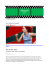More information

### Wedding MenuOutside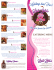More information

### Name: RAYMOND CRUZ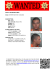More information

### Mise en page 1 - film press plus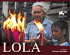More information

### Romantic Reads for Teens - Fairport Public Library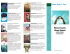More information

### A Boy and His Diva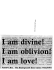More information

### LOLA JAMES HARPER EAU DE TOILETTE FRAGRANCES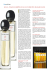More information

### TRANSFER PAT TERNS .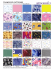More information